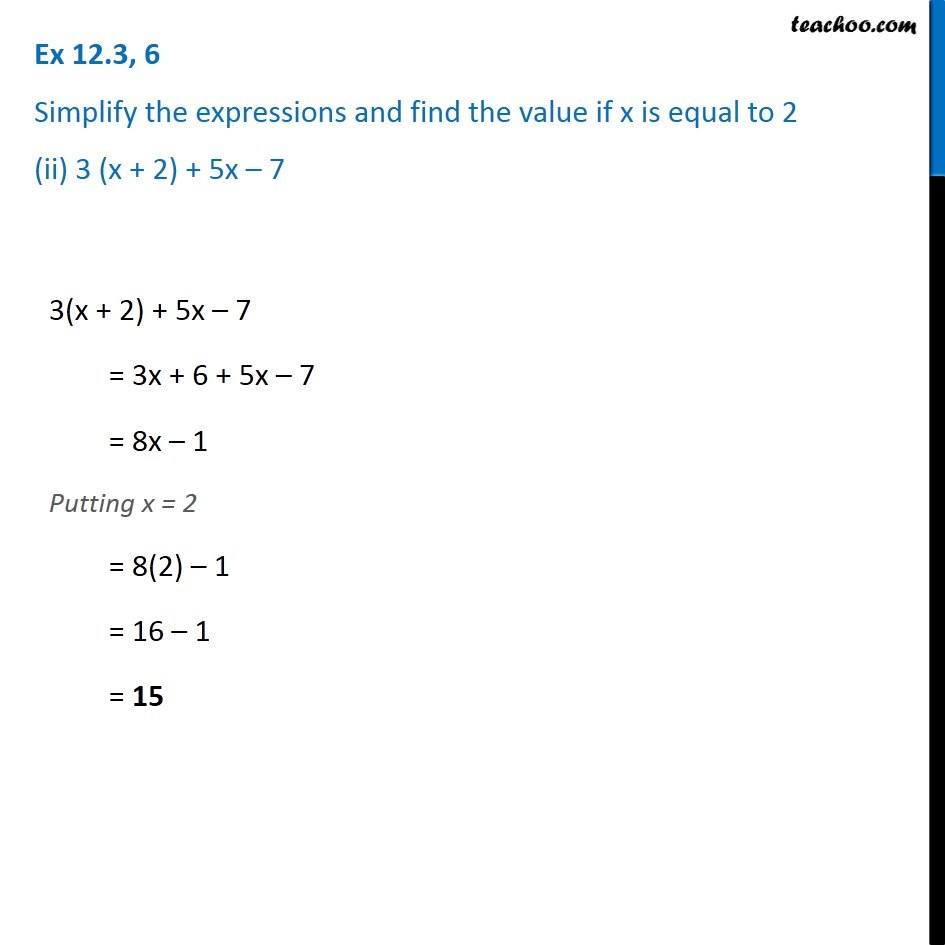1. Chapter 12 Class 7 Algebraic Expressions
2. Serial order wise
3. Ex 12.3

Transcript

Ex 12.3, 6 Simplify the expressions and find the value if x is equal to 2 (ii) 3 (x + 2) + 5x – 7 3(x + 2) + 5x – 7 = 3x + 6 + 5x – 7 = 8x – 1 Putting x = 2 = 8(2) – 1 = 16 – 1 = 15

Ex 12.3

Chapter 12 Class 7 Algebraic Expressions
Serial order wise